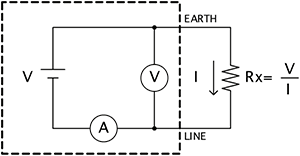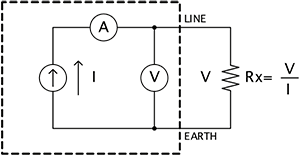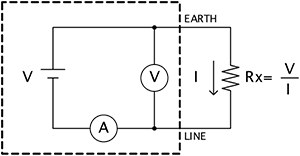# Insulation Tester Principles

## Principle of insulation-resistance measurement as implemented by insulation testers

The insulation resistance Rx of the measurement target is calculated by measuring the current I that flows to the target when the voltage V is applied and then dividing the applied voltage V by the resulting current I.## Principle of low-insulation-resistance measurement as implemented by insulation testers

The insulation resistance Rx of the measurement target is calculated by measuring the voltage V that occurs across the measurement terminals when the current I is applied to the target and then dividing the terminal voltage V by the applied current I.## Principle of photovoltaic resistance measurement as implemented by insulation testers

The resistance Rx of the measurement target is calculated by measuring the current I that flows to the target when the voltage V is applied and then dividing the applied voltage V by the resulting current I. (The voltage and current values produced by the measurement target as it generates electricity are subtracted.)